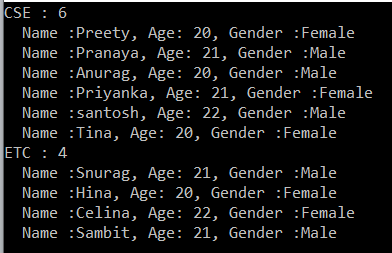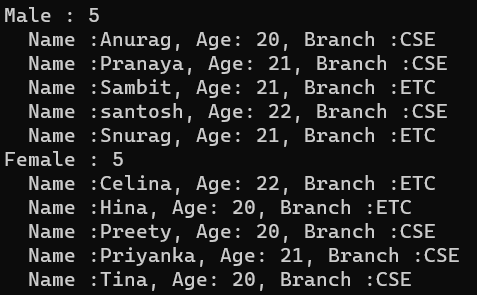# LINQ GroupBy Method in C#

## LINQ GroupBy Method in C# with Examples

In this article, I will discuss the LINQ GroupBy Method in C# with Examples. Please read our previous article discussing LINQ Deferred Execution vs Immediate Execution in C# with Examples. The LINQ GroupBy Method in C# belongs to the Grouping Operators Category. This method exactly does the same thing as the Group By clause does in SQL Query. As part of this article, we will discuss the following pointers related to the GroupBy Method in C#.

1. What is LINQ GroupBy Method in C#?
2. How to use the LINQ GroupBy Method using both Query and Method Syntax?
3. How to use the GroupBy Method and the OrderBy Method in C#?
4. Multiple Examples to Understand GroupBy Method in C#.
5. When to use the LINQ GroupBy Method in C#?
##### What is LINQ GroupBy Method in C#?

The GroupBy method in LINQ (Language Integrated Query) in C# is used for organizing and categorizing data into groups. It enables us to group elements in a collection based on a specified key and perform operations on each group.

GroupBy Method groups elements that share a common attribute, allowing us to perform operations on each group. Each group is represented by an IGrouping<TKey, TElement> object, where TKey is the type of the key, and TElement is the type of the elements in the group.

##### Examples to Understand LINQ GroupBy Method in C#

Let us understand how to use the LINQ GroupBy Method in C# using both Method and Query Syntax with Examples. We will use the following Student class to understand the GroupBy Method. So, create a class file named Student.cs and copy and paste the following code. This class has five properties: ID, Name, Gender, Branch, and Age. This class also has one method called GetStudents(), which returns a list of all students and will be our data source.

```using System.Collections.Generic;
namespace GroupByDemo
{
public class Student
{
public int ID { get; set; }
public string Name { get; set; }
public string Gender { get; set; }
public string Barnch { get; set; }
public int Age { get; set; }

public static List<Student> GetStudents()
{
return new List<Student>()
{
new Student { ID = 1001, Name = "Preety", Gender = "Female", Barnch = "CSE", Age = 20 },
new Student { ID = 1002, Name = "Snurag", Gender = "Male", Barnch = "ETC", Age = 21  },
new Student { ID = 1003, Name = "Pranaya", Gender = "Male", Barnch = "CSE", Age = 21  },
new Student { ID = 1004, Name = "Anurag", Gender = "Male", Barnch = "CSE", Age = 20  },
new Student { ID = 1005, Name = "Hina", Gender = "Female", Barnch = "ETC", Age = 20 },
new Student { ID = 1006, Name = "Priyanka", Gender = "Female", Barnch = "CSE", Age = 21 },
new Student { ID = 1007, Name = "santosh", Gender = "Male", Barnch = "CSE", Age = 22  },
new Student { ID = 1008, Name = "Tina", Gender = "Female", Barnch = "CSE", Age = 20  },
new Student { ID = 1009, Name = "Celina", Gender = "Female", Barnch = "ETC", Age = 22 },
new Student { ID = 1010, Name = "Sambit", Gender = "Male",Barnch = "ETC", Age = 21 }
};
}
}
}```
##### Grouping the Students Based on the Branch

Now, our requirement is to group the students based on Branch. For a better understanding, please look at the following example, which exactly does the same thing. The following example organizes the students into groups based on their branch (i.e., the branch will act as the key). It also means that students with the same branch will be stored in the same group, where each group has a key and the corresponding student collection. Here, the key will be the Branch, and the collection will be the student belonging to that branch. In the example below, I show how to use the GroupBy Method using both Method Syntax and Query Syntax.

```using System;
using System.Collections.Generic;
using System.Linq;

namespace GroupByDemo
{
class Program
{
static void Main(string[] args)
{
//Using Method Syntax
IEnumerable<IGrouping<string, Student>> GroupByMS = Student.GetStudents().GroupBy(s => s.Barnch);

//Using Query Syntax
IEnumerable<IGrouping<string, Student>> GroupByQS = (from std in Student.GetStudents()
group std by std.Barnch);

//It will iterate through each groups
foreach (IGrouping<string, Student> group in GroupByMS)
{
Console.WriteLine(group.Key + " : " + group.Count());

//Iterate through each student of a group
foreach (var student in group)
{
Console.WriteLine("  Name :" + student.Name + ", Age: " + student.Age + ", Gender :" + student.Gender);
}
}

}
}
}```
###### Output:Note: Each group has a key, and you can access the value of the key by using the key property. Along the same line, you can use the count property to check how many elements are in that group. Again, using a for each loop, you can access all the elements of a group, as shown in the above example.

##### Grouping Students by Gender in Descending Order, Names in Ascending Order in Each Group

Let us see an example to Understand the LINQ GroupBy Method with the following Requirements.

1. First, Grouping the Students by Gender.
2. Sort the Groups in Descending Order, i.e., soring the gender in Descending Order.
3. Finally, Sort the Student Names in each group in Ascending Order.

In the following example, we group the students by Gender using the LINQ GroupBy Method. Then, we sort the data by Gender in descending order, and finally, we sort the students in each group by their name in Ascending Order. The following example code is self-explained, so please go through the comment lines.

```using System;
using System.Linq;

namespace GroupByDemo
{
class Program
{
static void Main(string[] args)
{
//Using Method Syntax
//First Group the Data by Gender
var GroupByMS = Student.GetStudents().GroupBy(s => s.Gender)
//Then Sorting the data based on key in Descending Order
.OrderByDescending(c => c.Key)
.Select(std => new
{
Key = std.Key,
//Sorting the Students in Each Group based on Name in Ascending order
Students = std.OrderBy(x => x.Name)
});

//Using Query Syntax
//First Group the Data by Gender
var GroupByQS = from std in Student.GetStudents()
//First store the data into a group
group std by std.Gender into stdGroup
//Then Sorting the data based on key in Descending Order
orderby stdGroup.Key descending
select new
{
Key = stdGroup.Key,
//Sorting the Students in Each Group based on Name in Ascending order
Students = stdGroup.OrderBy(x => x.Name)
};

//It will iterate through each groups
foreach (var group in GroupByQS)
{
Console.WriteLine(group.Key + " : " + group.Students.Count());

//Iterate through each student of a group
foreach (var student in group.Students)
{
Console.WriteLine("  Name :" + student.Name + ", Age: " + student.Age + ", Branch :" + student.Barnch);
}
}

}
}
}```
###### Output:In the above example, the result is projected to an anonymous type. If you want, you create a new type with the required properties, and then you can project the result to that new type. Let us understand this. First, create a class file named StudentGroup.cs and copy and paste the following code.

```using System.Collections.Generic;
namespace GroupByDemo
{
public class StudentGroup
{
public string Key { get; set; }
public List<Student> Students { get; set; }
}
}```

With the above changes in place, now modify the Main method of the Program class as follows. Here, we are projecting the result into the newly created StudentGroup type instead of the anonymous one.

```using System;
using System.Linq;

namespace GroupByDemo
{
class Program
{
static void Main(string[] args)
{
//Using Method Syntax
//First Group the Data by Gender
var GroupByMS = Student.GetStudents().GroupBy(s => s.Gender)
//Then Sorting the data based on key in Descending Order
.OrderByDescending(c => c.Key)
.Select(std => new StudentGroup
{
Key = std.Key,
//Sorting the Students in Each Group based on Name in Ascending order
Students = std.OrderBy(x => x.Name).ToList()
});

//Using Query Syntax
//First Group the Data by Gender
var GroupByQS = from std in Student.GetStudents()
//First store the data into a group
group std by std.Gender into stdGroup
//Then Sorting the data based on key in Descending Order
orderby stdGroup.Key descending
select new StudentGroup
{
Key = stdGroup.Key,
//Sorting the Students in Each Group based on Name in Ascending order
Students = stdGroup.OrderBy(x => x.Name).ToList()
};

//It will iterate through each groups
foreach (var group in GroupByQS)
{
Console.WriteLine(group.Key + " : " + group.Students.Count());

//Iterate through each student of a group
foreach (var student in group.Students)
{
Console.WriteLine("  Name :" + student.Name + ", Age: " + student.Age + ", Branch :" + student.Barnch);
}
}

}
}
}```
###### Output:##### When to use the LINQ GroupBy Method in C#?

Understanding when to use the GroupBy method can significantly enhance the efficiency and readability of your code. Here are some common scenarios where GroupBy is particularly useful:

• Categorizing Data: When you have a collection of items and need to categorize or classify them into groups based on one or more attributes, GroupBy is an ideal choice. For instance, grouping a list of employees by department or categorizing products by category.
• Data Aggregation: GroupBy is extremely useful when you need to perform aggregate operations (like count, sum, average, min, max) on subsets of data. For example, calculating the total sales per region or finding the average salary per department.
• Data Summarization: Use GroupBy when you need to summarize data to create reports or to provide a summarized view of the data. It can help generate summary tables where data is grouped along one dimension and various aggregates are calculated.
• Hierarchical Data Presentation: When presenting data in a hierarchical structure, such as in tree views or grouped lists, GroupBy can be used to create the top-level groups under which data will be nested.
• Eliminating Duplicate Records: In scenarios where you need to eliminate duplicates based on certain criteria, you can group by the criteria and select one item from each group. This is especially useful in scenarios where you don’t have a straightforward key to distinguish.
• Complex Queries in Entity Framework: When working with Entity Framework or similar ORMs, GroupBy can be used to translate complex queries to the database level, especially when dealing with large datasets, to improve performance by reducing the amount of data transferred to the application.

In the next article, I will discuss the LINQ GroupBy Method with Multiple Keys in C# with Examples. In this article, I try to explain the LINQ GroupBy Methid in C# with Examples. I hope you understand the need and how to use the LINQ Groupby Method in C# and the OrderBy Method with Examples.